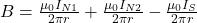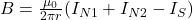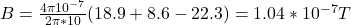## Three long, straight electrical cables, running north and south, are tightly enclosed in an insulating sheath. One of the cables carries a 2

Question

Three long, straight electrical cables, running north and south, are tightly enclosed in an insulating sheath. One of the cables carries a 22.3 A current southward; the other two carry currents of 18.9 A and 8.6 A northward.
Use Ampere’s law to calculate the magnitude of the magnetic field at a distance of 10.0 \rm m from the cables.

in progress 0
2 months 2021-07-24T05:27:39+00:00 2 Answers 2 views 0

The resultant magnetic field is -1.04×10⁻⁷T

Explanation:

Given:

i₁ = current through the first wire = 22.3 A (south)

i₂ = current through the second wire = 18.9 A (north)

i₃ = current through the third wire = 8.6 A (north)

R = distance from the cables = 10 m

The magnetic field through the first wire is equal: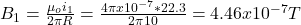The magnetic field through the second wire is equal: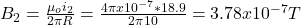The magnetic field through the third wire is equal: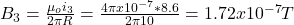The resultant magnetic field is equal: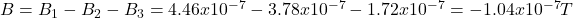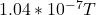Explanation:

By using Ampere’s law formula we have: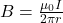where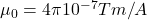is the permeability constant. r = 10 m is the distance from the wire, and I is the current magnitude. As there are 2 wires carrying currents northward and 1 carrying current southward: